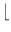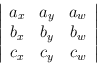Next: Rational Segments ( rat_segment Up: Basic Data Types for Previous: Iso-oriented Rectangles ( rectangle   Contents   Index

# Rational Points ( rat_point )

Definition

An instance of data type rat_point is a point with rational coordinates in the two-dimensional plane. A point with cartesian coordinates (a, b) is represented by homogeneous coordinates (x, y, w) of arbitrary length integers (see Integers of Arbitrary Length) such that a = x/w and b = y/w and w > 0.

#include < LEDA/geo/rat_point.h >

Types

 rat_point::coord_type the coordinate type (rational). rat_point::point_type the point type (rat_point). rat_point::float_type the corresponding floating-point type (point).

Creation

 rat_point p introduces a variable p of type rat_point initialized to the point (0, 0). rat_point p(const rational& a, const rational& b) introduces a variable p of type rat_point initialized to the point (a, b). rat_point p(integer a, integer b) introduces a variable p of type rat_point initialized to the point (a, b). rat_point p(integer x, integer y, integer w) introduces a variable p of type rat_point initialized to the point with homogeneous coordinates (x, y, w) if w > 0 and to point (- x, - y, - w) if w < 0. Precondition w0. rat_point p(const rat_vector& v) introduces a variable p of type rat_point initialized to the point (v, v). Precondition: v.dim() = 2. rat_point p(const point& p1, int prec = rat_point::default_precision) introduces a variable p of type rat_point initialized to the point with homogeneous coordinates (P*x,P*y, P), where p1 = (x, y) and P = 2prec. If prec is non-positive, the conversion is without loss of precision, i.e., P is chosen as a sufficiently large power of two such that P*x and P*y are integers. rat_point p(double x, double y, int prec = rat_point::default_precision) see constructor above with p = (x, y).

Operations

 point p.to_float() returns a floating point approximation of p. rat_vector p.to_vector() returns the vector extending from the origin to p. void p.normalize() simplifies the homogenous representation by dividing all coordinates by gcd (X, Y, W). integer p.X() returns the first homogeneous coordinate of p. integer p.Y() returns the second homogeneous coordinate of p. integer p.W() returns the third homogeneous coordinate of p. double p.XD() returns a floating point approximation of p.X(). double p.YD() returns a floating point approximation of p.Y(). double p.WD() returns a floating point approximation of p.W(). rational p.xcoord() returns the x-coordinate of p. rational p.ycoord() returns the y-coordinate of p. double p.xcoordD() returns a floating point approximation of p.xcoord(). double p.ycoordD() returns a floating point approximation of p.ycoord(). rat_point p.rotate90(const rat_point& q, int i = 1) returns p rotated by i x 90 degrees about q. If i > 0 the rotation is counter-clockwise otherwise it is clockwise. rat_point p.rotate90(int i = 1) returns p rotated by i x 90 degrees about the origin. If i > 0 the rotation is counter-clockwise otherwise it is clockwise. rat_point p.reflect(const rat_point& p, const rat_point& q) returns p reflected across the straight line passing through p and q. Precondition pq. rat_point p.reflect(const rat_point& q) returns p reflected across point q. rat_point p.translate(const rational& dx, const rational& dy) returns p translated by vector (dx, dy). rat_point p.translate(integer dx, integer dy, integer dw) returns p translated by vector (dx/dw, dy/dw). rat_point p.translate(const rat_vector& v) returns p + v, i.e., p translated by vector v. Precondition v.dim() = 2. rat_point p + const rat_vector& v returns p translated by vector v. rat_point p - const rat_vector& v returns p translated by vector - v. rational p.sqr_dist(const rat_point& q) returns the squared distance between p and q. int p.cmp_dist(const rat_point& q, const rat_point& r) returns compare(p.sqrdist(q), p.sqrdist(r)). rational p.xdist(const rat_point& q) returns the horizontal distance between p and q. rational p.ydist(const rat_point& q) returns the vertical distance between p and q. int p.orientation(const rat_point& q, const rat_point& r) returns orientation(p, q, r) (see below). rational p.area(const rat_point& q, const rat_point& r) returns area(p, q, r) (see below). rat_vector p - const rat_point& q returns the difference vector of the coordinates.

Non-Member Functions

 int cmp_signed_dist(const rat_point& a, const rat_point& b, const rat_point& c, const rat_point& d) compares (signed) distances of c and d to the straight line passing through a and b (directed from a to b). Returns +1 (-1) if c has larger (smaller) distance than d and 0 if distances are equal. int orientation(const rat_point& a, const rat_point& b, const rat_point& c) computes the orientation of points a, b, c as the sign of the determinanti.e., it returns +1 if point c lies left of the directed line through a and b, 0 if a,b, and c are collinear, and -1 otherwise. int cmp_distances(const rat_point& p1, const rat_point& p2, const rat_point& p3, const rat_point& p4) compares the distances (p1,p2) and (p3,p4). Returns +1 (-1) if distance (p1,p2) is larger (smaller) than distance (p3,p4), otherwise 0. rat_point midpoint(const rat_point& a, const rat_point& b) returns the midpoint of a and b. rational area(const rat_point& a, const rat_point& b, const rat_point& c) computes the signed area of the triangle determined by a,b,c, positive if orientation(a, b, c) > 0 and negative otherwise. bool collinear(const rat_point& a, const rat_point& b, const rat_point& c) returns true if points a, b, c are collinear, i.e., orientation(a, b, c) = 0, and false otherwise. bool right_turn(const rat_point& a, const rat_point& b, const rat_point& c) returns true if points a, b, c form a righ turn, i.e., orientation(a, b, c) < 0, and false otherwise. bool left_turn(const rat_point& a, const rat_point& b, const rat_point& c) returns true if points a, b, c form a left turn, i.e., orientation(a, b, c) > 0, and false otherwise. int side_of_halfspace(const rat_point& a, const rat_point& b, const rat_point& c) returns the sign of the scalar product (b - a)*(c - a). If ba this amounts to: Let h be the open halfspace orthogonal to the vector b - a, containing b, and having a in its boundary. Returns +1 if c is contained in h, returns 0 is c lies on the the boundary of h, and returns -1 is c is contained in the interior of the complement of h. int side_of_circle(const rat_point& a, const rat_point& b, const rat_point& c, const rat_point& d) returns +1 if point d lies left of the directed circle through points a, b, and c, 0 if a,b,c,and d are cocircular, and -1 otherwise. bool incircle(const rat_point& a, const rat_point& b, const rat_point& c, const rat_point& d) returns true if point d lies in the interior of the circle through points a, b, and c, and false otherwise. bool outcircle(const rat_point& a, const rat_point& b, const rat_point& c, const rat_point& d) returns true if point d lies outside of the circle through points a, b, and c, and false otherwise. bool on_circle(const rat_point& a, const rat_point& b, const rat_point& c, const rat_point& d) returns true if points a, b, c, and d are cocircular. bool cocircular(const rat_point& a, const rat_point& b, const rat_point& c, const rat_point& d) returns true if points a, b, c, and d are cocircular. int compare_by_angle(const rat_point& a, const rat_point& b, const rat_point& c, const rat_point& d) compares vectors b-a and d-c by angle (more efficient than calling vector::compare_by_angle(b-a,d-x) on rat_vectors). bool affinely_independent(const array& A) decides whether the points in A are affinely independent. bool contained_in_simplex(const array& A, const rat_point& p) determines whether p is contained in the simplex spanned by the points in A. A may consist of up to 3 points. Precondition The points in A are affinely independent. bool contained_in_affine_hull(const array& A, const rat_point& p) determines whether p is contained in the affine hull of the points in A.Next: Rational Segments ( rat_segment Up: Basic Data Types for Previous: Iso-oriented Rectangles ( rectangle   Contents   Index